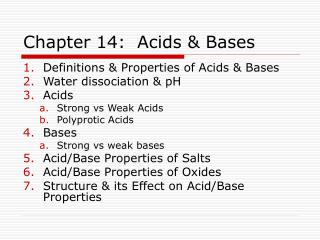DownloadDownload PresentationChapter 14: Acids & Bases

# Chapter 14: Acids & Bases

Download Presentation## Chapter 14: Acids & Bases

- - - - - - - - - - - - - - - - - - - - - - - - - - - E N D - - - - - - - - - - - - - - - - - - - - - - - - - - -
##### Presentation Transcript

1. Chapter 14: Acids & Bases • Definitions & Properties of Acids & Bases • Water dissociation & pH • Acids • Strong vs Weak Acids • Polyprotic Acids • Bases • Strong vs weak bases • Acid/Base Properties of Salts • Acid/Base Properties of Oxides • Structure & its Effect on Acid/Base Properties

2. Example 1 Write dissociation reactions for the following acids: HC2H3O2 C2H5NH3+ Fe(H2O)63+ HClO4

3. Example 2 Find the concentrations of H+, OH-, and Cl– in a solution of 0.30 M HCl. What is the pH of this solution?

4. Example 3 Find the concentrations of all species present, the pH, and the percent dissociation of a 0.12 M solution of formic acid, HCHO2.

5. Example 4 Find the pH of a solution that is 1.0 M in hydrocyanic acid and 1.0 M in hydrofluoric acid.

6. Example 5 Calculate Ka for a 0.055M solution of propionic acid, HC3H5O2 that has a pH of 3.06.

7. Example 6 Calculate Ka for a 0.109M solution of formic acid, HCHO2 that has a percent ionization of 3.7%.

8. Example 7 Calculate the concentrations of H+ and CO32- in a 5.45 x 10-4 M solution of carbonic acid.

9. Example 8 H2SeO4 is a strong acid with a Ka2 of 1.2 x 10-2. • Find the pH of a 0.150 M solution of H2SeO4 if the second H+ is ignored. • Find the pH of a 0.150 M solution of H2SeO4 if the second H+ is included.

10. Example 9 Find the concentrations of H+ and OH- in a solution of 0.30 M KOH. Find the pH of this solution.

11. Example 10 Find [OH-] and the pH of a 0.15 M solution of hydroxylamine, NH2OH. Kb = 1.1 x 10-8

12. Example 11 The pH of a 0.63M solution of methylamine is 12.22 at 25°C. What is Kb for methylamine at this temperature?

13. Example 12 Calculate Kb for the cyanide ion.

14. Example 13 Find Kb for the sulfate ion.

15. Example 14 Find Ka for the ammonium ion.

16. Example 15 Predict whether each of the following solutions is acidic, basic, or neutral. • HONH3Cl (aq) • K3PO4 (aq) • Cu(NO3)2 (aq) • CH3NH3OCl (aq)

17. Example 16 Household bleach is 0.80 M NaClO. Find the concentrations of OH- and ClO- and the pH of household bleach.

18. Example 17 Find the pH of a solution that is 0.10 M CH3NH3OCl.

19. Example 18 Find the pH of a solution that is 0.10 M H3PO4.

20. Example 19 Find the pH of a solution that is 0.10 M NaH2PO4.

21. Example 20 Find the pH of a solution that is 0.10 M K2HPO4.

22. Ka Values: Monoprotic Acids

23. Ka Values: Polyprotic Acids

24. Kb Values: